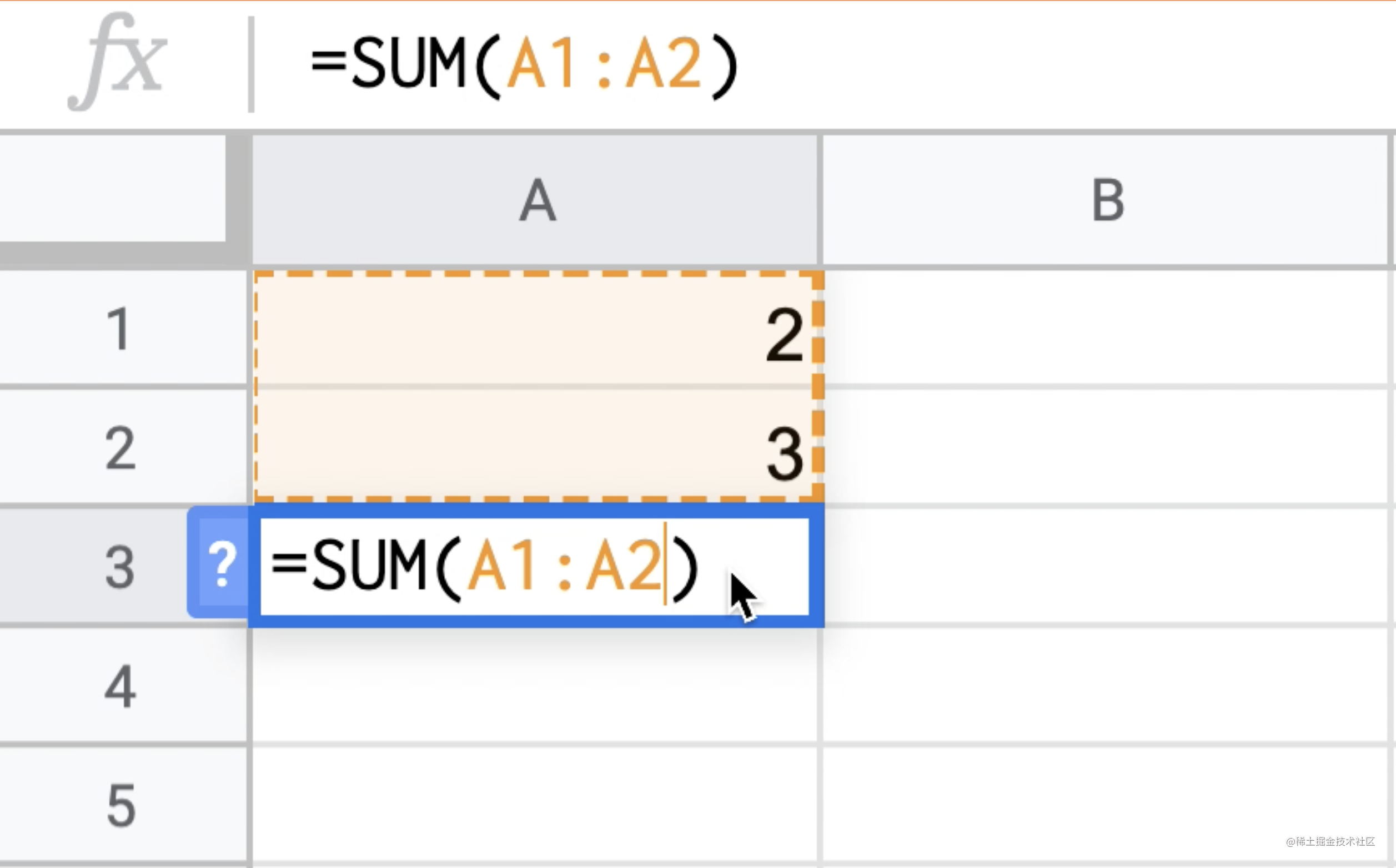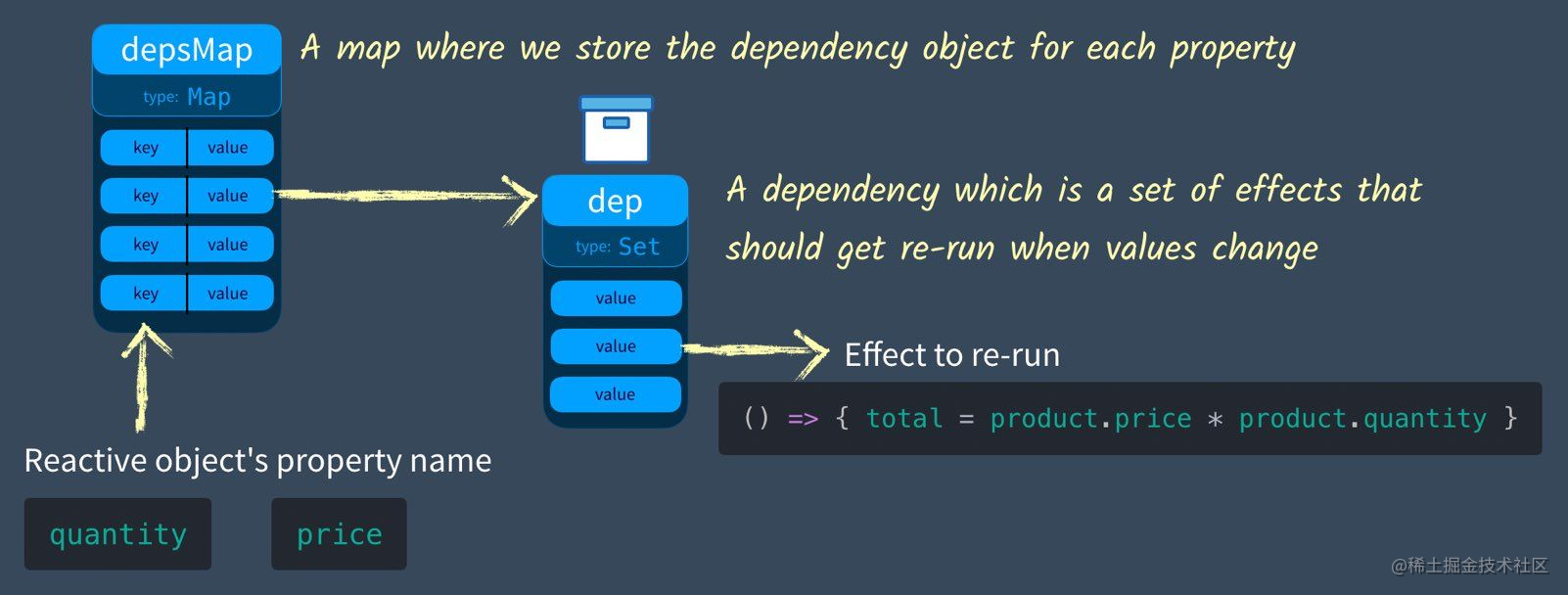Vue3.0源码解析「reactive」篇 — 0.Reactivity 基本概念0.Reactivity 基本概念

What is reactivity?In React

class List extends React.Component {
constructor() {
this.state = {
price: 5.00,
quantity: 2,
};
}
this.setState('quantity', this.quantity+1);
}
render() {
return (
<ul>
<li>price: {this.state.price}</li>
<li>sum: {this.state.price * this.state.quanity}</li>
</ul>
)
}
}

In Vue

<div id="app">
<div>Price: \${{ product.price }}</div>
<div>Total: \${{ product.price * product.quantity }}</div>
<div>Taxes: \${{ totalPriceWithTax }}</div>
</div>
<script src="https://cdn.jsdelivr.net/npm/vue"></script>
<script>
var vm = new Vue({
el: '#app',
data: {
product: {
price: 5.00,
quantity: 2
}
},
computed: {
totalPriceWithTax() {
return this.product.price * this.product.quantity * 1.03
}
}
})
</script>

• 更新页面上 price 价格的显示。
• 重新计算price乘以quantity的表达式，并更新页面。
• 再次调用 totalPriceWithTax 函数并更新页面。

let product = { price: 5, quantity: 2 }
let total = product.price * product.quantity  // 10 right?
product.price = 20
console.log(`total is \${total}`)

Track And Trigger Effects

• effect：副作用，即某个响应式对象属性或其本身发生变化之后，对其他个体产生的影响。
• Track：跟踪，跟踪即不断记录某个响应式对象属性的副作用。
• Trigger：触发，当某个对象本身/属性发生变化之后，我们需要触发之前 track 的所有 effect

Effect & Track & Trigger

let product = { price: 5, quantity: 2 }
let total = 0

let effect = () => { total = product.price * product.quantity }

effect() // Also go ahead and run it

track()  // Remember this in case we want to run it later

let dep = new Set() // Our object tracking a list of effects

function track () {
dep.add(effect) // Store the current effect
}

function trigger() {
dep.forEach(effect => effect())
}

let product = { price: 5, quantity: 2 }
let total = 0
let dep = new Set()

function track() {
}

function trigger() {
dep.forEach(effect => effect())
}

let effect = () => {
total = product.price * product.quantity
}

track()
trigger()

product.price = 20
console.log(total) // => 10

trigger()
console.log(total) // => 40

Multiple Properties现在我们每次将属性值改变之后调用 tracktrigger都需要一个新参数就是属性名，上面的代码将会这么被改写：

const depsMap = new Map()
function track(key, effect) {
// Make sure this effect is being tracked.
let dep = depsMap.get(key) // Get the current dep (effects) that need to be run when this key (property) is set
if (!dep) {
// There is no dep (effects) on this key yet
depsMap.set(key, (dep = new Set())) // Create a new Set
}
}
}
function trigger(key) {
let dep = depsMap.get(key) // Get the dep (effects) associated with this key
if (dep) { // If they exist
dep.forEach(effect => {
// run them all
effect()
})
}
}

Multiple Reactive Objects

const targetMap = new WeakMap()
// targetMap stores the effects that each object should re-run when it's updated

function track(target, key) {
// We need to make sure this effect is being tracked.
let depsMap = targetMap.get(target) // Get the current depsMap for this target

if (!depsMap) {
// There is no map.
targetMap.set(target, (depsMap = new Map())) // Create one
}

let dep = depsMap.get(key) // Get the current dependencies (effects) that need to be run when this is set
if (!dep) {
// There is no dependencies (effects)
depsMap.set(key, (dep = new Set())) // Create a new Set
}

}

function trigger(target, key) {
const depsMap = targetMap.get(target) // Does this object have any properties that have dependencies (effects)
if (!depsMap) {
return
}

let dep = depsMap.get(key) // If there are dependencies (effects) associated with this
if (dep) {
dep.forEach(effect => {
// run them all
effect()
})
}
}

True Reactivity In Vue3

• GET property => 获取属性的时候我们需要 track 这个获取操作产生的 effect
• SET property => 我们需要为此属性触发任何跟踪的依赖项(effects)。

Vue 3 中使用 ES6 Proxy and Reflect 来拦截 GETSET 调用。之前的 Vue 2 使用 Object.defineProperty 完成这个操作。下面来讲解一下具体的实现：

Proxy & Reflect

const proxyObj = new Proxy(obj, {
},
},
deleteProperty(target, key) {
return Reflect.deleteProperty(target, key);
},
});

Proxy 支持我们在使用、设置、删除对象的时候进行拦截，一般和 Reflect 联用，注意 Reflect 存在的意义只是对对象属性操作规范化以及函数式编程的支持，Reflectapi 会返回操作结果。

- target[key] = val
+ Reflect.deleteProperty(target, key)
- delete target.key

Combina proxy with Track&Trigger

const targetMap = new WeakMap()
// targetMap stores the effects that each object should re-run when it's updated
function track(target, key) {
// We need to make sure this effect is being tracked.
let depsMap = targetMap.get(target) // Get the current depsMap for this target
if (!depsMap) {
// There is no map.
targetMap.set(target, (depsMap = new Map())) // Create one
}
let dep = depsMap.get(key)
// Get the current dependencies (effects) that need to be run when this is set
if (!dep) {
// There is no dependencies (effects)
depsMap.set(key, (dep = new Set()))
// Create a new Set
}
// Add effect to dependency map
}

function trigger(target, key) {
const depsMap = targetMap.get(target)
// Does this object have any properties that have dependencies (effects)
if (!depsMap) {
return
}
let dep = depsMap.get(key)
// If there are dependencies (effects) associated with this
if (dep) {
dep.forEach(effect => {
// run them all
effect()
})
}
}

function reactive(target) {
const handler = {
let result = Reflect.get(target, key, receiver)
track(target, key)
// If this reactive property (target) is GET inside then track the effect to rerun on SET
return result
},
let oldValue = target[key]
let result = Reflect.set(target, key, value, receiver)
if (result && oldValue != value) {
trigger(target, key)
// If this reactive property (target) has effects to rerun on SET, trigger them.
}
return result
}
}
return new Proxy(target, handler)
}

let product = reactive({ price: 5, quantity: 2 })
let total = 0

let effect = () => {
total = product.price * product.quantity
}
effect()

console.log('before updated quantity total = ' + total) // 10
product.quantity = 3
console.log('after updated quantity total = ' + total)  // 15

Nested reactivity

const handler = {
track(target, property)
const value = Reflect.get(...arguments)
if (isObject(value)) {
// 将嵌套对象包裹在自己的响应式代理中
return reactive(value)
} else {
return value
}
}
// ...
}